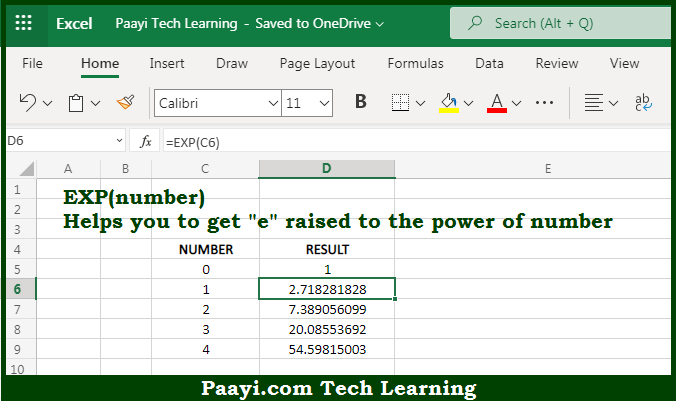# Learn How to Use Microsoft Excel EXP Function

Written by | 0 Comments | 527 Views

In this article, you will learn how to use the Microsoft Excel EXP function and its prime function in Microsoft Excel. You will also get to know the Microsoft Excel EXP function return value and syntax with the help of some examples.

Microsoft Excel EXP Function

The main function of the Microsoft Excel EXP function is used to find the value of "e" raised to the power of provided number. So, with the help of the EXP function, you can able to return the result of the constant "e" raised to the power of a number. It should be noted that the constant "e" is a numeric constant that relates to the exponential growth and decay whose value is approximately 2.71828. The EXP function is the inverse of the LN, which is the Natural Logarithm function. So, with the help of the Microsoft Excel EXP function, you can easily find the value of "e" raised to the power of provided number.

Return Value of EXP Function

The return value will be the constant "e" raised to the power of a number.

Syntax of EXP Function

=EXP(number)

Where the arguments:

• number: This is the power that "e" is raised to.

How to Use Microsoft Excel EXP Function?So we know that the Microsoft Excel EXP function is used to find the value of "e" raised to the power of provided number, where e ≈ 2.718. So, with the help of the EXP function, you can able to return the result of the constant "e" raised to the power of a number. It should be noted that the constant "e" is a numeric constant that relates to the exponential growth and decay whose value is approximately 2.71828. The EXP function is the inverse of the LN, which is the Natural Logarithm function. So, with the help of the Microsoft Excel EXP function, you can easily the value of "e" raised to the power of provided number.